# How to count the number of Sigma and Pi bonds?

Home  > Chemistry > How to count sigma and pi bonds?

Covalent bonds in organic compounds are divided into two types, the essential, strong bond that is difficult to break, and the weak bond that is easy to break, the essential bond is the sigma bond (σ), and the other is the pi bond (𝛑).

In this article, we will learn how to count sigma and pi bonds, by defining and differentiating them, and we will also learn how to count the sigma bond and the pi bond in a Lewis structure, double bonds, and triple bonds.

Page Contents

## What are the Sigma and Pi bonds?

### Sigma bond

It is the only bond in the single covalent bonds, and it is symbolized by the Greek letter sigma (σ). A Sigma bond is formed when two atoms share electrons and their valence levels directly overlap (end to end) as shown in the figure.

It exists when the s orbital overlaps with another s orbital, a p orbital with another py orbital, or a p orbital with an s orbital as in hydrogen molecule H2, ammonia NH3, and fluorine F2.### Pi bond

The pi bond is found in multiple covalent bonds i.e. double covalent bonds which consist of one sigma bond and one pi bond, and triple covalent bonds which consist of one sigma bond and two pi bonds.

The pi bond is denoted by the Greek letter (𝛑) and arises from the overlapping of parallel p orbitals as shown in the figure given below.To be able to count the sigma and pi bonds in any compound remember that:

 ❖    The sigma bond is the strongest bond, and it is formed before the pi bond. ❖    The single bond is the sigma bond. (1 single bond = 1 sigma bond) ❖    The double bond contains one Sigma bond and one pi bond. (1 double bond = 1 sigma bond + 1 pi bond) ❖    The triple bond contains a sigma bond and two pi bonds. (1 triple bond = 1 sigma bond + 2 pi bonds) ❖    The sigma bond is present in all chemical compounds. In contrast, the pi bond is found in compounds that contain double or triple bonds such as alkenes, alkynes, carboxyl groups, aldehydes, ketones, carbonyl groups, and others.

## How to count sigma and pi bonds?

 Using two steps, you can count the number of sigma and pi bonds for any compound.(1). Draw the Lewis structure of the compound.(2). Determine the type of the bond (Single, Double, or Triple bond).All single bonds are sigma bonds. (Single bond = 1 sigma bond).A double bond contains a sigma bond and a pi bond. (Double bond = 1 sigma + 1 pi bond).The triple bond contains one sigma bond and two pi bonds. (Triple bond = 1 sigma + 2 pi bonds).For example – If the molecule has 3 single bonds and 1 double bond, that means, we have 4 sigma bonds and 1 pi bond. [∴ 3 single bond means 3 sigma bond and 1 double bond means 1 sigma + 1 pi bond].

Let’s see some examples and learn how to count sigma and pi bonds:

 Count the sigma and pi bonds in CH4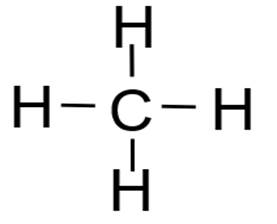From the Lewis structure of CH4, we note that all bonds in the compound are single, meaning that all are sigma bonds.∴ Thus, the CH4 compound contains four sigma bonds and zero pi bonds.
 Count the sigma and pi bonds in Ethane (C2H6)First, we draw the Lewis structure of the Ethane (C2H6) compound: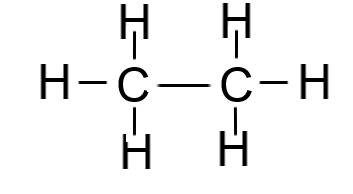From the Lewis diagram, we see, all bonds of the compound (C2H6) are single and therefore, all are sigma bonds.∴ So, Ethane (C2H6) has 7 sigma bonds and zero pi bonds.
 Count the sigma and pi bonds in NH3First, we draw the Lewis structure of the Ammonia (NH3) compound: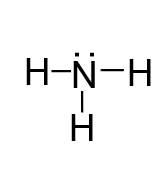All bonds are single, so they all are sigma bonds.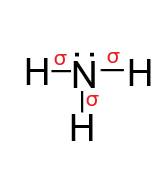∴ So, Ammonia contains 3 sigma bonds and zero pi bonds.

## How many sigma and pi bonds are in a double bond?

Remember, A double bond contains 1 sigma and 1 pi bond. (1 double bond = 1 sigma bond + 1 pi bond)

Examples of compounds that contain double bonds.

 Count the sigma and pi bonds in ethene (C2H4):The compound shows that it contains both single bonds and double bonds, all single bonds are sigma bonds, and the double bond contains a sigma bond and a pi bond.Ethene has 4 single bonds and 1 double bond.4 single bonds mean 4 sigma bonds and 1 double bond means (1 sigma + 1 pi bond).So, In ethene (C2H4), the number of sigma bonds is 5, and the pi bond is only 1.
 Count the sigma and pi bonds in Acetaldehyde (CH3CHO)At first, glance, when looking at the compound, we do not find the double bond, but in fact, the double bond is present in the aldehyde group (CHO) as shown by its structure,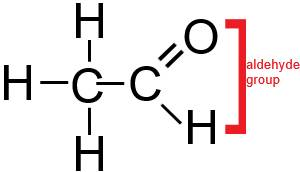According to the above figure, there are five single bonds and one double bond in CH3CHO.5 single bond means 5 sigma bond and 1 double means (1 sigma + 1 pi bond).So, in acetaldehyde (CH3CHO), the number of sigma bonds is 6 and the pi bond is only 1.Count the sigma and pi bonds in Acetone (CH3COCH3)As in the previous example, the chemical formula does not show the presence of a double bond, but attention should be paid to the presence of a ketone group in the compound as shown by its structure.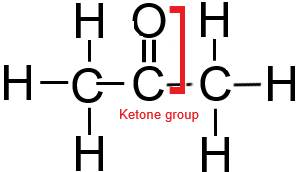According to the above figure, there are eight single bonds and one double bond in CH3COCH3.8 single bond means 8 sigma bond and 1 double means (1 sigma + 1 pi bond).So, in acetone (CH3COCH3), the number of sigma bonds is 9 and the pi bond is only 1.## How many sigma and pi bonds are in triple bonds?

A triple bond contains one sigma bond and two pi bonds, the sigma bond is in the middle between the two pi bonds. (1 triple bond = 1 sigma bond + 2 pi bonds).

Examples of compounds that contain triple bonds:

 Count the sigma and pi bonds in the Nitrogen molecule (N2)Nitrogen (N2) molecule contains a triple bond between two nitrogen atoms.So, “the triple bond between the two nitrogen atoms comprises one sigma bond and two pi bonds.”
 Count the sigma and pi bonds in COAlso, the compound (CO) contains only a triple bond, i.e. one sigma bond and two pi bonds.In the CO molecule, one sigma and two pi bonds are present.
 Count the sigma and pi bonds in Butyne (C4H6)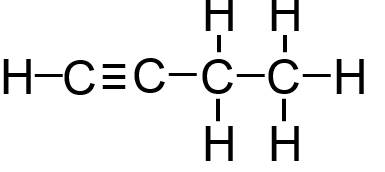According to the above figure, there are eight single bonds and one triple bond in C4H6.8 single bond means 8 sigma bond and 1 triple means (1 sigma + 2 pi bond).So, in Butyne (C4H6), the number of sigma bonds is 9 and the pi bond is 2.

## More examples: Count the sigma and pi bonds in a compound

 Calculate the number of sigma and pi bonds in Hexane (C6H14)According to the above figure, there are 19 single bonds present.So, in Hexane (C6H12), the number of sigma bonds is 19.
 Calculate the number of sigma and pi bonds in CH3OCH3According to the above figure, there are 8 single bonds present.So, in CH3OCH3, the number of sigma bonds is 8.
 Calculate the number of sigma and pi bonds in CO32-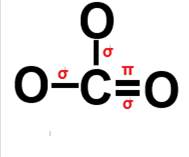According to the above figure, there are 2 single bonds and 1 double bond-.2 single bond means 2 sigma bond and 1 double bond means (1 sigma + 1 pi bond).So, in CO32-, the number of sigma bonds is 3 and the pi bond is only 1.

## Difference between sigma and pi bonds?

Here, some important difference between sigma and pi bonds.

 Comparison Sigma bond (σ) Pi bond (𝛑) Kind of interference Directly (end to end) Side-by-side Force Strong Weaker Overlapping orbitals p+p, p+s, s+s p+p Electron density Distributed in the interference region along the line connecting the two nuclei Distributed in the overlapping region on the upper and lower sides of the interconnected orbits Counting A sigma bond is found in single as well as multiple covalent bonds. (1 single bond = 1 sigma bond) A pi bond is found only in multiple covalent bonds. (1 double bond = 1 sigma bond + 1 pi bond), (1 triple bond = 1 sigma bond + 2 pi bonds).

Note: For determining sigma and pi bonds in the compound, it is very useful to learn the drawing process of lewis structure.

Also check –

## FAQ

### How many sigma and bonds are present in benzene (C6H6)?

As we learned earlier, all double bonds contain one pi bond and one sigma bond.

In benzene, we notice that there are 3 double bonds, so undoubtedly we have 3 pi bonds + 3 sigma bonds. Also, we see, 9 single bonds also here, so 9 sigma bonds. So when we add we get 12 sigma and 3 pi bonds in Benzene.So, in Benzene (C6H6), the number of sigma bonds is 12, and the pi bond is 3.

### How many pi bonds are present in ethane?

We know, that ethane is an alkane and that alkanes are saturated, that is, it contains the maximum possible number of hydrogen atoms, and therefore it does not contain double and triple bonds.

Since the pi bond is found only in double and triple bonds, then ethane does not contain the pi bond.### How many sigma and bonds are present in Caffeine (C8H10N4O2)?From the Lewis structure of caffeine, we find that it contains 4 double bonds, so 4 pi bonds, and the sum of the sigma bonds in the single and double bonds is equal to 25 sigma bonds.

So, in Caffeine (C8H10N4O2), the number of sigma bonds is 25, and the pi bond is 4.

### How many sigma and pi bonds are in Ethyne (C2H2)?• By looking at the above lewis diagram, we see, Ethyne contains 2 single bonds and 1 triple bond.
• 2 single bond means 2 sigma bonds, and one triple bond means 1 sigma + 2 pi bond.
• Therefore, in ethyne (C2H2), a total of 3 sigmas and 2 pi bonds are present.### How many sigma and pi bonds are in Water (H2O)?• By looking at the above lewis diagram, we see, Water contains only 2 single bonds.
• 2 single bonds mean 2 sigma bonds
• Therefore, in water (H2O), a total of 2 sigmas bonds are present. There is no pi bond in H2O since it doesn’t contain any multiple bonds.## Summary

• To count the sigma and pi bonds, all you need to remember is that, (1 single bond = 1 sigma bond), (1 double bond = 1 sigma bond + 1 pi bond), (1 triple bond = 1 sigma bond + 2 pi bonds).
• The Sigma bond is represented by (σ) and the pi bond is represented by (𝛑).
• A pi bond is found in multiple covalent bonds i.e. double covalent bonds which consist of one sigma bond and one pi bond, and triple covalent bonds which consist of one sigma bond and two pi bonds.
• The Sigma bond is stronger than the pi bond.
Did you like it?

#### Vishal Goyal

Vishal Goyal is the founder of Topblogtenz, a comprehensive resource for students seeking guidance and support in their chemistry studies. He holds a degree in B.Tech (Chemical Engineering) and has four years of experience as a chemistry tutor. The team at Topblogtenz includes experts like experienced researchers, professors, and educators, with the goal of making complex subjects like chemistry accessible and understandable for all. A passion for sharing knowledge and a love for chemistry and science drives the team behind the website. Let's connect through LinkedIn: https://www.linkedin.com/in/vishal-goyal-2926a122b/

Share it...